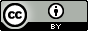# Liu, S.; Cai, S.; Cao, C.; Li, Z. Molecular Electronegative Distance Vector (MEDV) Related to 15 Properties of Alkanes. J. Chem. Inf. Model. 2000, 40, 6, 1337–1348.

## QsarDB content

### Property BP: Boiling point [K]

##### Model_16: Table 6A

Regression model (regression)

Open in:QDB Explorer QDB Predictor

Name Type n

R2

σ

Training set training 150 0.996 3.234

### Property D: Density at 25C [g/cm^3]

##### Model_17: Table 6B

Regression model (regression)

Open in:QDB Explorer QDB Predictor

Name Type n

R2

σ

Training set training 134 0.988 2.874

### Property MR: Molar refraction at 20C [m^3/mol]

##### Model_7: Table 6C

Regression model (regression)

Open in:QDB Explorer QDB Predictor

Name Type n

R2

σ

Training set training 69 1.000 0.065

### Property RI: Refraction index at 25C

##### Model_18: Table 6D

Regression model (regression)

Open in:QDB Explorer QDB Predictor

Name Type n

R2

σ

Training set training 134 0.977 0.002

### Property CT: Critical temperature [K]

##### Model_19: Table 6E

Regression model (regression)

Open in:QDB Explorer QDB Predictor

Name Type n

R2

σ

Training set training 74 0.991 5.357

### Property CP: Critical pressure [atm]

##### Model_20: Table 6F

Regression model (regression)

Open in:QDB Explorer QDB Predictor

Name Type n

R2

σ

Training set training 74 0.982 0.582

### Property ST: Surface tension at 20C [dyn/cm]

##### Model_21: Table 6G

Regression model (regression)

Open in:QDB Explorer QDB Predictor

Name Type n

R2

σ

Training set training 68 0.982 0.262

### Property MV: Molar volume at 20C [cm3/mol]

##### Model_8: Table 6H

Regression model (regression)

Open in:QDB Explorer QDB Predictor

Name Type n

R2

σ

Training set training 69 1.000 0.362

### Property HC: Heat capacity at 300K [J*K^-1*mol^-1]

##### Model_9: Table 6I

Regression model (regression)

Open in:QDB Explorer QDB Predictor

Name Type n

R2

σ

Training set training 134 0.977 3.822

### Property E: Enthalphy at 300K

##### Model_10: Table 6J

Regression model (regression)

Open in:QDB Explorer QDB Predictor

Name Type n

R2

σ

Training set training 134 0.987 0.483

### Property HV: Heat of vaporization at 25C [kJ/mol]

##### Model_11: Table 6K

Regression model (regression)

Open in:QDB Explorer QDB Predictor

Name Type n

R2

σ

Training set training 69 0.998 0.225

### Property HA: Heat of atomization at 25C [kJ/mol]

##### Model_12: Table 6L

Regression model (regression)

Open in:QDB Explorer QDB Predictor

Name Type n

R2

σ

Training set training 44 1.000 30.330

### Property HF: Standard heat of formation at 25C [kJ/mol]

##### Model_13: Table 6M

Regression model (regression)

Open in:QDB Explorer QDB Predictor

Name Type n

R2

σ

Training set training 54 0.992 2.604

### Property HFL: Heat of formation in liquid at 25C [kJ/mol]

##### Model_14: Table 6N

Regression model (regression)

Open in:QDB Explorer QDB Predictor

Name Type n

R2

σ

Training set training 62 0.995 0.727

### Property HFG: Heat of formation in gas at 25C [kJ/mol]

##### Model_15: Table 6O

Regression model (regression)

Open in:QDB Explorer QDB Predictor

Name Type n

R2

σ

Training set training 63 0.995 0.705

## Citing

When using this QDB archive, please cite (see details) it together with the original article:

• Piir, G. Data for: Molecular Electronegative Distance Vector (MEDV) Related to 15 Properties of Alkanes. QsarDB repository, QDB.122. 2014. http://dx.doi.org/10.15152/QDB.122

• Liu, S.; Cai, S.; Cao, C.; Li, Z. Molecular Electronegative Distance Vector (MEDV) Related to 15 Properties of Alkanes. J. Chem. Inf. Model. 2000, 40, 1337–1348. http://dx.doi.org/10.1021/ci0003247

 Title: Liu, S.; Cai, S.; Cao, C.; Li, Z. Molecular Electronegative Distance Vector (MEDV) Related to 15 Properties of Alkanes. J. Chem. Inf. Model. 2000, 40, 6, 1337–1348. Abstract: Several quantitative structure−property relationship (QSPR) models between 15 basic physical properties or thermodynamic functions of alkanes and their molecular electronegative distance vectors (MEDV) are developed. For six of the properties boiling point (BP), density (D) at 25 °C, refraction index (RI) at 25 °C, critical temperature (CT), critical pressure (CP), and surface tension (ST) at 20 °C logarithmic models are found to give better results than conventional (linear) models since the values of these properties all tend to a limit with increasing carbon chain length. All models are created using multiple linear regression (MLR). Conventional models are proposed for the remaining nine physical properties or thermodynamic functions:  molar volume (MV) at 20 °C, molar refraction (MR) at 20 °C, heat capacity (HC) at 300 K, enthalpy (E) at 300 K, heats of vaporization (HV) at 25 °C, heat of atomization (HA) at 25 °C, standard heat of formation (HF) at 25 °C, heat of formation in liquid (HFL) at 25 °C, and heat of formation in gas (HFG) at 25 °C. URI: http://hdl.handle.net/10967/122 http://dx.doi.org/10.15152/QDB.122 Date: 2014-10-30
﻿

## Files in this item

Name Description Format Size View
2000JCICS1337.zip QSPR models for 15 physico-chemical properties of alkanes application/zip 143.2Kb View/OpenFiles associated with this item are distributed